#For years the students of Singapore have scored higher than those of any other country on standardized math and science tests (administered by Trends in International Mathematics and Science Study). Primary Mathematics was the standard curriculum for elementary students in Singapore and continues to gain popularity in the U.S. due to its proven effectiveness. The Earlybird Kindergarten and New Elementary Math (grades 7-8) series are recommended by the U.S. publisher of Primary Mathematics.

While these courses were designed for a formal classroom setting, they adapt easily for home education. The material requires teacher explanation (especially in the lower grades), but extensive online help is available, and the teacher's guides contain supplementary material not found in the student texts. English is the primary instructional language in Singapore, so you don't have to worry about awkward translations that make no sense. Although the metric system is taught primarily, there is mention of English measurement; discussions of money are suited to U.S. currency. Overall, the cultural barrier for American users is minimal and by no means insurmountable.

## Preschool & Kindergarten

The Earlybird Kindergarten series for 4- and 5-year-olds introduces very basic concepts like counting and numeral identification in a colorful format; teacher's guides are not necessary, though useful to get the most out of this program. Essential Math Kindergarten is similar in approach, a little less teacher-oriented and faster moving than Earlybird, but also less visually engaging for the child (it's also less expensive).

Primary Mathematics for grades 1-6 is the course responsible for securing Singapore students their stellar test scores. The series follows a ConcretePictorialAbstract approach designed to make sense of math to students with a variety of learning styles. The concrete and pictorial stages introduce new concepts in real-life contexts, while the abstract stage provides the thinking skills necessary to apply those ideas in ways the text doesn't address. Adult supervision and independent practice go hand in hand as students put into action what they learn. Mental math is introduced early so kids learn to actually think mathematically, not just relying on manipulatives and their fingers to solve problems. Currently the U.S. and Standards editions are both available; the newer Standards series meets the California Board of Education requirements, but either option is appropriate for most families or schools.

What is the difference between the US and Standards Editions?

Honestly, there's not much difference between the two. The Standards series definitely has a few perks, such as full color textbooks throughout (US textbooks are only full-color through grade 2), test books, and slightly expanded workbooks and "Extra Practice" books. Standards includes a few new chapters or sections on data analysis and probability, plane and solid figures, negative numbers, and coordinate graphing. But the content change is minimal, mostly just rearranged with topics being introduced slightly earlier in Standards.

Standards is more expensive, to the tune of \$8-16 per year just for the texts, workbooks and home instructor's guides. Although it adds Test books, it loses the Intensive Practice books, workbooks that review the concepts taught but increase the difficulty of the problems. The current editions of Challenging Word Problems can be used with either edition.

If you desire a more in-depth comparison, please read our reviews of the US and Standards editions, look at this comparative scope & sequence, or visit this FAQ page.

## Junior & Senior High

In Singapore, the term elementary refers to the basic elements of higher math, like algebra, geometry, trigonometry, etc., and there are several options generally covering grades 7-10. There are also two basic "streams": "normal stream" is moderately paced and teaches the bare essentials, while "express stream" is fast paced and intended for students who wish to pursue advanced math studies. Click on the links below for expanded reviews, links to FAQs, and a comparison chart.

• The New Elementary Mathematics books ("express stream"), like the Primary Math series, emphasize critical thinking and problem solving skills. The texts are fast-paced and for advanced students. Singapore Math has discontinued the books for grades 9 and 10, so this series currently stops at eighth grade.
• Dimensions Mathematics (formerly Discovering Mathematics) is a "normal stream" course designed to be largely student-directed; this is also available only through 8th grade.

## Our Honest Opinion:

We prefer to promote things that lots of people like. While there are those out there who don't like Singapore math, it's kind of hard to argue with a whole nation of kids who consistently excel at math as the result of a proven program. Primary Math is that program, though New Elementary Math largely follows the same principles in its approach to math instruction.

Kids are encouraged not to simply memorize a bunch of math facts (some of that is involved), but to actually think mathematically. While many courses promise to get kids thinking in this way, Singapore's programs seem like some of the few that actually accomplish the goal. The idea is not for kids simply to be able to complete limited operations under prescribed circumstances, but to give them the tools they need in order to be able to figure problems out on their own. These books move considerably faster than comparable curricula, but the intent is to teach conceptually, not just a series of rules.

Some kids can't keep up with the content in these books, though that isn't a common complaint. Primary Math is probably a better place to start than with the New Elementary series because it lays the foundations for creative critical thinking. When you listen to different math curriculum publishers, the idea of getting kids to think mathematically doesn't seem unique; but as far as actually getting kids to think mathematically, Singapore does seem unique.

None of these are geared to independent learning—all of them are teacher-dependent (though for Primary Math, teacher-intensive would be a stretch). These are fairly easy to adapt to a home school setting, and are excellent not only for teaching math but also for teaching logic and problem-solving skills. While some courses might be more fun (or not), Singapore is unbeatable when it comes to good solid instruction and learning.

Please Note: SingaporeMath.com asks us not to sell large quantities to public schools and school districts. We are happy to fill orders from private schools, individual teachers, and home school families, but if you are ordering for a school district, please contact Singapore directly through their website. Singapore Math® is a registered trademark of SingaporeMath.com, Inc. Used with permission.Review by C. Hollis Crossman C. Hollis Crossman used to be a child. Now he is a husband and father, teaches adult Sunday school in his Presbyterian congregation, and likes weird stuff. He might be a mythical creature, but he's definitely not a centaur.Read more of his reviews here.

Did you find this review helpful?
Parent Categories
Singapore Math®
Singapore Math®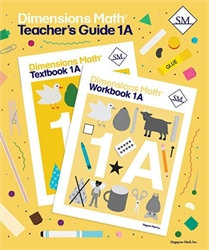Dimensions Math 1A - Teacher's Guide from Singapore Math for 1st grade in Dimensions Mathematics (Location: MAT-SMDM)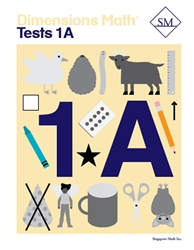Dimensions Math 1A - Tests from Singapore Math for 1st grade in Dimensions Mathematics (Location: MAT-SMDM)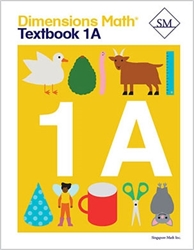Dimensions Math 1A - Textbook from Singapore Math for 1st grade in Dimensions Mathematics (Location: MAT-SMDM) \$12.00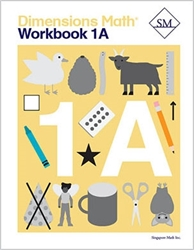Dimensions Math 1A - Workbook from Singapore Math for 1st grade in Dimensions Mathematics (Location: MAT-SMDM)Dimensions Math 1B - Teacher's Guide from Singapore Math for 1st grade in Dimensions Mathematics (Location: MAT-SMDM)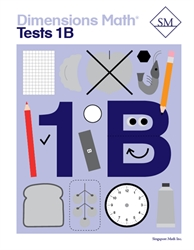Dimensions Math 1B - Tests from Singapore Math for 1st grade in Dimensions Mathematics (Location: MAT-SMDM)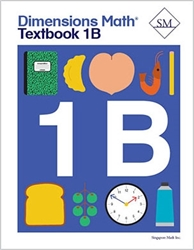Dimensions Math 1B - Textbook from Singapore Math for 1st grade in Dimensions Mathematics (Location: MAT-SMDM) \$12.00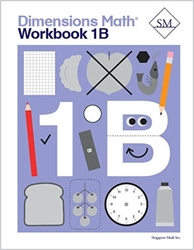Dimensions Math 1B - Workbook from Singapore Math for 1st grade in Dimensions Mathematics (Location: MAT-SMDM) \$12.00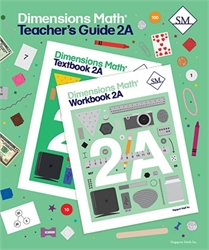Dimensions Math 2A - Teacher's Guide from Singapore Math for 2nd grade in Dimensions Mathematics (Location: MAT-SMDM)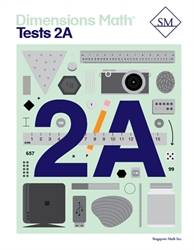Dimensions Math 2A - Tests from Singapore Math for 2nd grade in Dimensions Mathematics (Location: MAT-SMDM)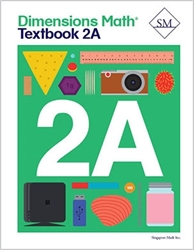Dimensions Math 2A - Textbook from Singapore Math for 2nd grade in Dimensions Mathematics (Location: MAT-SMDM)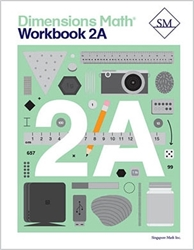Dimensions Math 2A - Workbook from Singapore Math for 2nd grade in Dimensions Mathematics (Location: MAT-SMDM) \$12.00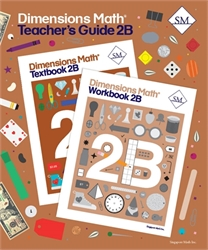Dimensions Math 2B - Teacher's Guide from Singapore Math for 2nd grade in Dimensions Mathematics (Location: MAT-SMDM)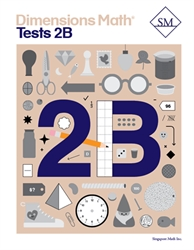Dimensions Math 2B - Tests from Singapore Math for 2nd grade in Dimensions Mathematics (Location: MAT-SMDM)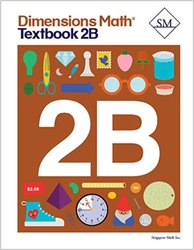Dimensions Math 2B - Textbook from Singapore Math for 2nd grade in Dimensions Mathematics (Location: MAT-SMDM) \$12.00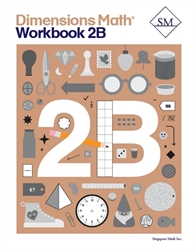Dimensions Math 2B - Workbook from Singapore Math for 2nd grade in Dimensions Mathematics (Location: MAT-SMDM) \$12.00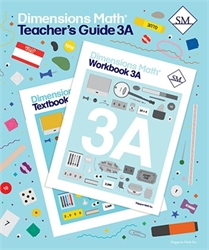Dimensions Math 3A - Teacher's Guide from Singapore Math for 3rd grade in Dimensions Mathematics (Location: MAT-SMDM)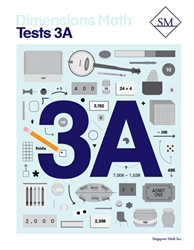Dimensions Math 3A - Tests from Singapore Math for 3rd grade in Dimensions Mathematics (Location: MAT-SMDM) \$12.00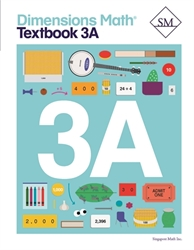Dimensions Math 3A - Textbook from Singapore Math for 3rd grade in Dimensions Mathematics (Location: MAT-SMDM) \$12.00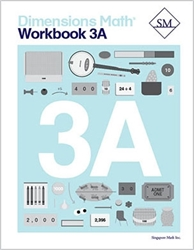Dimensions Math 3A - Workbook from Singapore Math for 3rd grade in Dimensions Mathematics (Location: MAT-SMDM) \$12.00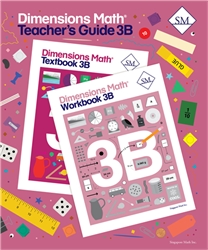Dimensions Math 3B - Teacher's Guide from Singapore Math for 3rd grade in Dimensions Mathematics (Location: MAT-SMDM) \$25.00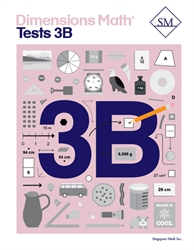Dimensions Math 3B - Tests from Singapore Math for 3rd grade in Dimensions Mathematics (Location: MAT-SMDM) \$12.00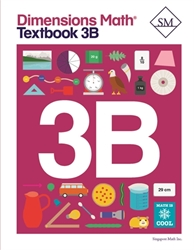Dimensions Math 3B - Textbook from Singapore Math for 3rd grade in Dimensions Mathematics (Location: MAT-SMDM) \$12.00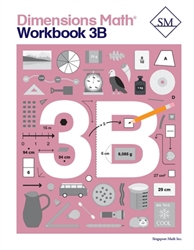Dimensions Math 3B - Workbook from Singapore Math for 3rd grade in Dimensions Mathematics (Location: MAT-SMDM) \$12.00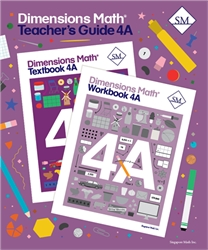Dimensions Math 4A - Teacher's Guide from Singapore Math for 4th grade in Dimensions Mathematics (Location: MAT-SMDM)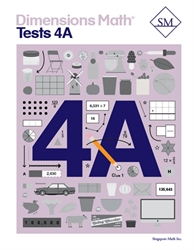Dimensions Math 4A - Tests from Singapore Math for 4th grade in Dimensions Mathematics (Location: MAT-SMDM) \$12.00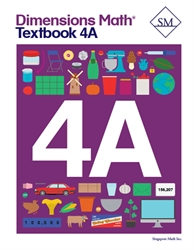Dimensions Math 4A - Textbook from Singapore Math for 4th grade in Dimensions Mathematics (Location: MAT-SMDM) \$12.00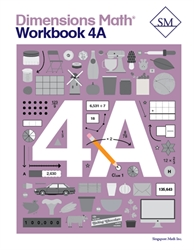Dimensions Math 4A - Workbook from Singapore Math for 4th grade in Dimensions Mathematics (Location: MAT-SMDM) \$12.00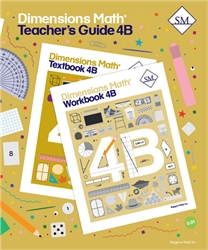Dimensions Math 4B - Teacher's Guide from Singapore Math for 4th grade in Dimensions Mathematics (Location: MAT-SMDM)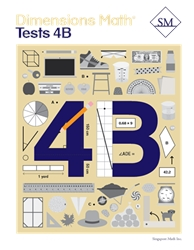Dimensions Math 4B - Tests from Singapore Math for 4th grade in Dimensions Mathematics (Location: MAT-SMDM) \$12.00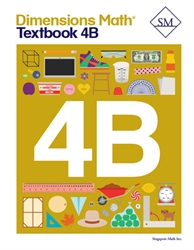Dimensions Math 4B - Textbook from Singapore Math for 4th grade in Dimensions Mathematics (Location: MAT-SMDM) \$12.00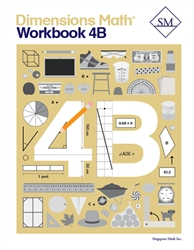Dimensions Math 4B - Workbook from Singapore Math for 4th grade in Dimensions Mathematics (Location: MAT-SMDM) \$12.00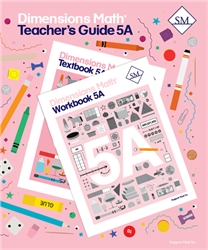Dimensions Math 5A - Teacher's Guide (2020) from Singapore Math for 5th grade in Dimensions Mathematics (Location: MAT-SMDM) Dimensions Math 5A - Tests (2020?) from Singapore Math for 5th grade in Dimensions Mathematics (Location: MAT-SMDM)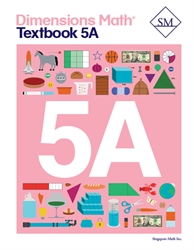Dimensions Math 5A - Textbook from Singapore Math for 5th grade in Dimensions Mathematics (Location: MAT-SMDM) \$12.00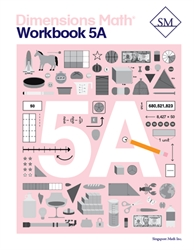Dimensions Math 5A - Workbook from Singapore Math for 5th grade in Dimensions Mathematics (Location: MAT-SMDM) \$12.00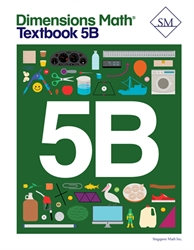Dimensions Math 5B - Textbook from Singapore Math for 5th grade in Dimensions Mathematics (Location: MAT-SMDM) \$12.00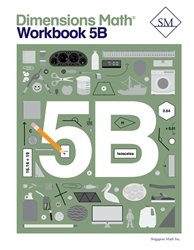Dimensions Math 5B - Workbook (July 2020) from Singapore Math for 5th grade in Dimensions Mathematics (Location: MAT-SMDM)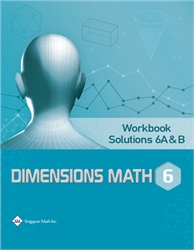Dimensions Math 6A & 6B - Workbook Solutions from Star Publishing for 6th grade in Dimensions Mathematics (Location: MAT-SMDM) \$10.00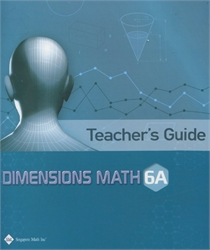Dimensions Math 6A - Teacher's Guide from Star Publishing for 8th grade in Dimensions Mathematics (Location: MAT-SMDM)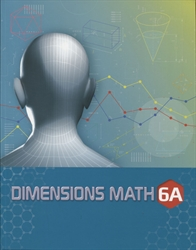Dimensions Math 6A - Textbook from Star Publishing for 6th grade in Dimensions Mathematics (Location: MAT-SMDM) \$22.50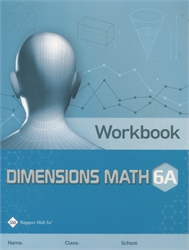Dimensions Math 6A - Workbook from Star Publishing for 8th grade in Dimensions Mathematics (Location: MAT-SMDM) \$12.00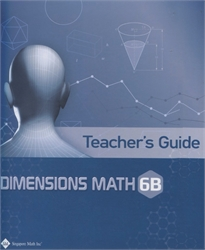Dimensions Math 6B - Teacher's Guide from Star Publishing for 8th grade in Dimensions Mathematics (Location: MAT-SMDM) \$29.00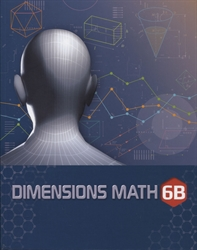Dimensions Math 6B - Textbook from Star Publishing for 8th grade in Dimensions Mathematics (Location: MAT-SMDM) \$22.50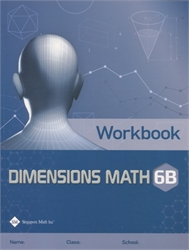Dimensions Math 6B - Workbook from Star Publishing for 8th grade in Dimensions Mathematics (Location: MAT-SMDM) \$12.00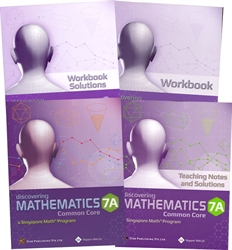Dimensions Math 7A - Bundle for 7th grade in Dimensions Mathematics (Location: MAT-SMDM) \$82.50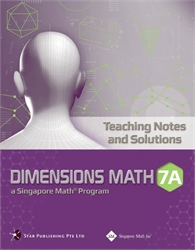Dimensions Math 7A - Teaching Notes & Solutions from Star Publishing for 7th grade in Dimensions Mathematics (Location: MAT-SMDM) \$31.00 \$22.00 (2 in stock)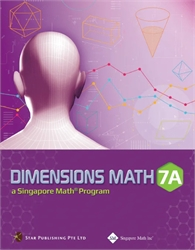Dimensions Math 7A - Textbook from Singapore Math for 7th grade in Dimensions Mathematics (Location: MAT-SMDM) \$24.00 \$16.00 (1 in stock)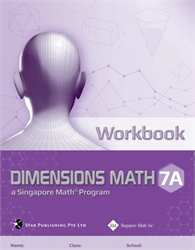Dimensions Math 7A - Workbook from Singapore Math for 7th grade in Dimensions Mathematics (Location: MAT-SMDM) \$11.00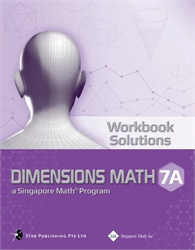Dimensions Math 7A - Workbook Solutions from Singapore Math for 7th grade in Dimensions Mathematics (Location: MAT-SMDM) \$16.50